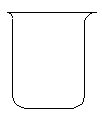The IO₃⁻/I₂ Catalyzed Decomposition of H₂O₂

Chemical Concept Demonstrated

• Catalytic decomposition

Demonstration

 The beaker contains the following solutions: Starch Solution Solution A: KIO3 and H2SO4 dissolved in water. Solution B: malonic acid and MnSO4 dissolved in water. Solution C: 30% H2O2 dissolved in water.Observations

The color sequence goes from deep blue to colorless to yellow to gold to brown to deep blue again.

Explanation (including important chemical equations)

The first primary step in this reaction is the oxidation of hydrogen peroxide by iodic acid:

5 H2O2 (aq) + 2 HIO3 (aq) --> 5 O2 (g) + I2 (aq) + 6 H2O (l)

The second step is the oxidation of of iodine by hydrogen peroxide:

5 H2O2 (aq) + I2 (aq) --> 2 HIO3 (aq) + 4 H2O (l)

The overall result is a decomposition of H2O2 into H2O and O2. The higher the initial concentrations, the shorter the induction period.

Contributors